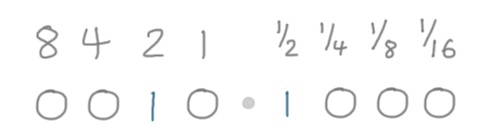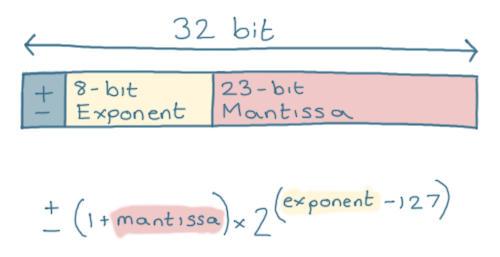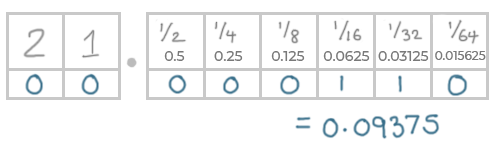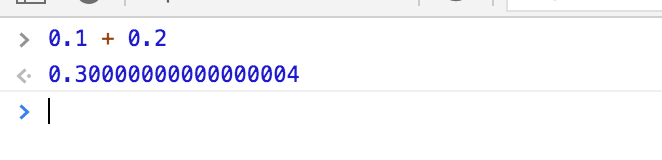Lorem ipsum dolor sit amet, consectetur adipiscing elit, sed do eiusmod tempor incididunt ut labore.

15 St Margarets, NY 10033
(+381) 11 123 4567
ouroffice@aware.com

### KMF#### What Are Floating-point Numbers? – DZone Web Dev

(originally posted to the BaseClass newsletter.)

## Why Should I Care?

Have you ever wanted to know:

• Why `0.1` + `0.3` does not always equal `0.4`?
• How we store non-integer numbers in binary?

## In 5 Minutes or Less:

You may be familiar with how we represent a number in binary.

Each bit represents a power of 2, and by combining them we can produce every whole number:

But what happens when we need to represent something that isn’t an integer, like `2.5`?

Let’s split the 8 bits in half.

We’ll use the ‘left’ half to represent the number before the decimal point (`2` in our example), and the ‘right’ half to represent the fraction after the decimal point (`0.5`, or ½ in this case).

In this system, `2.5` would be represented as `0 0 1 0 1 0 0 0`:We can represent fractions now, but we’ve lost a lot of range.

We can’t represent `16.0` in this format, for example. There just aren’t enough bits on the left of the point.

We could just keep adding more bits to store larger numbers, but this format is still quite limited.

Sometimes we want to store very large numbers, in which case we’d like more bits on the left. Other times, we’d like to store very small fractions, in which case we would need fewer bits on the left and more precision on the right.

This is what floating-point is; a way of storing numbers that allows the point to move to represent a larger range of values.

The standard for float values is called ‘IEEE 754’, which defines both 32 and 64-bit floating-point (or ‘float’) values.

Each 32 or 64-bit float is split into 3 sections.

• The first bit represents the ‘sign’; `0` for a positive number, or `1` for a negative number.
• The next 8 bits are called the ‘Exponent.’
• The final 23 bits are the ‘Mantissa.’

We use these 3 values in a formula, which gives us the number that the float represents:You don’t need to understand this formula, just know that this way of storing numbers means we can represent a much larger range.

By making the ‘exponent’ value larger or smaller we can represent very small fractions or very large numbers.

That’s why it’s called ‘floating-point,’ it doesn’t have a fixed point like our original example. Instead, the point ‘floats’, or moves, depending on the size of the ‘exponent.’

### Rounding Errors

There are some numbers we can’t represent exactly using our standard decimal system.

For example, we can’t represent ⅓ exactly in decimal:

`⅓ = 0.3333333...`

This is also true of some numbers in binary; they cannot be represented exactly.

For example, let’s try to represent `0.1` in binary.

Again, the values after the decimal point represent the fractions:This is very close to `0.1` – and it’s the best we can do in this example – but it is not exactly `0.1`.

The fact that our point can ‘move’ means that we can add more precision to this fraction if we like, but the fact remains that we just cannot represent some numbers exactly in binary.

This can lead to some interesting rounding errors.

If you try to calculate `0.2` + `0.1` in JavaScript, you’ll get an answer of `0.30000000000000004`:This is very close to the correct answer, but it’s not exactly the correct answer.

With this in mind, we usually compare floating-point numbers by checking the difference between them.

For example, instead of checking whether two numbers are equal, we would check that the difference between them is very small.

``Math.abs(result1 - result2) < 0.001``

This is a simplified example, of course. In reality, you would choose a tolerance appropriate to the calculation you are doing.

### Want to Know More?